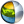Cg 3.1 Toolkit Documentation Cg / Standard Library / distance

## Name

distance - return the Euclidean distance between two points

## Synopsis

```float distance(float pt1, float pt2);
float distance(float1 pt1, float1 pt2);
float distance(float2 pt1, float2 pt2);
float distance(float3 pt1, float3 pt2);
float distance(float4 pt1, float4 pt2);

half distance(half pt1, half pt2);
half distance(half1 pt1, half1 pt2);
half distance(half2 pt1, half2 pt2);
half distance(half3 pt1, half3 pt2);
half distance(half4 pt1, half4 pt2);

fixed distance(fixed pt1, fixed pt2);
fixed distance(fixed1 pt1, fixed1 pt2);
fixed distance(fixed2 pt1, fixed2 pt2);
fixed distance(fixed3 pt1, fixed3 pt2);
fixed distance(fixed4 pt1, fixed4 pt2);
```

pt1
First point.
pt2
Second point.

## Description

Returns the Euclidean distance from a first point pt1 to a second point pt2.

## Reference Implementation

distance for a float3 vector could be implemented like this.

```float distance(float3 pt1, float3 pt2)
{
float3 v = vt2 - pt1;
return sqrt(dot(v,v));
}
```

## Profile Support

distance is supported in all profiles except fp20.| Cg Toolkit | Download | Release Archive | Profiles | Reference | Books | Discussions |